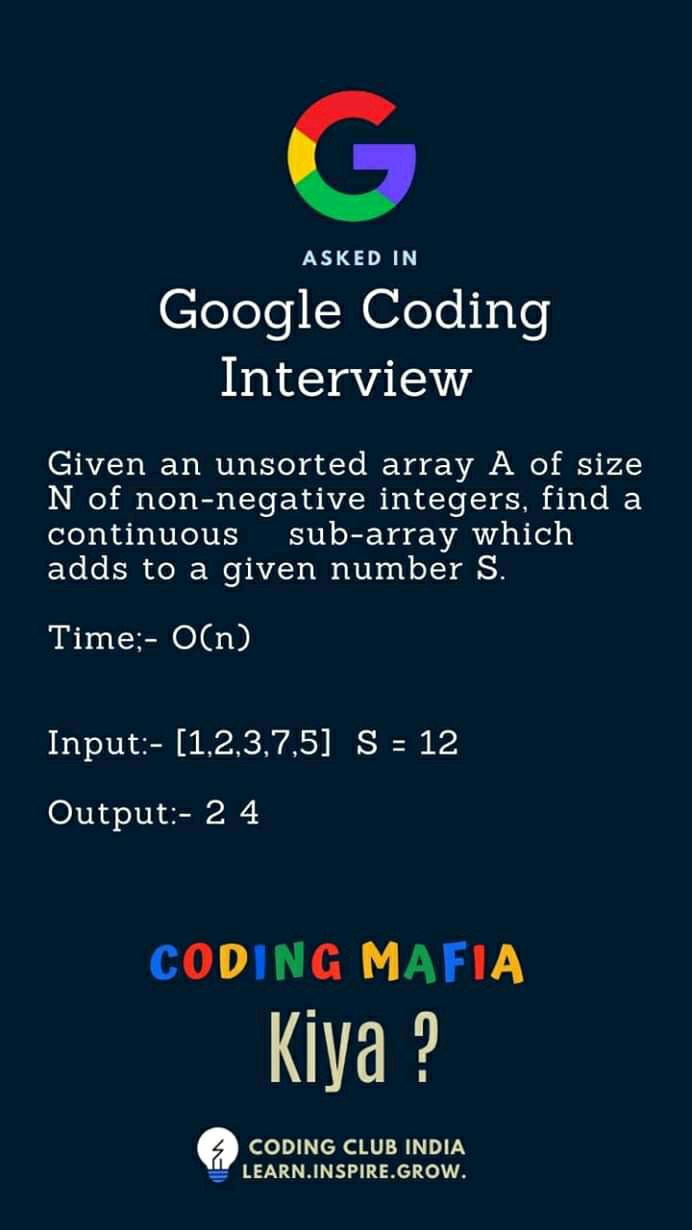## 题面## 哈希表法

$S = s_i - s_j(j \leq i)$

$s_i - S = s_j(j \leq i)$

 1 2 3 4 5 6 7  for(int i = 1;i<=n;++i) { s[i] = s[i - 1] + a[i]; if(map[s[i] - S] != 0) return make_pair(map[s[i] - S] + 1,i); map[s[i]] = i; } 

## 双指针扫描法

$S = s_i - s_j(j \leq i)$

  1 2 3 4 5 6 7 8 9 10  int lp = 0,rp = 0; while(lp <= rp && lp >= 0 && rp <= n) { if(s[rp] - s[lp] == S) return make_pair(lp + 1,rp); if(s[rp] - s[lp] < S) ++rp; else ++lp; } 

## 双指针扫描法证明

1. 初始时，不考虑空数组的情况，从 $L = 0,R = 1$ 开始，若成立则算法退出，否则命题成立。
2. 假定 $\forall a \in [0,L],b \in [L+1,R]$ 中命题已成立，
欲证 $\forall a \in [0,L+1],b \in [L+2,R]$ 中命题成立，
若 $s_{R} - s_{L+1} = S$ 则算法结束，因此要证明命题，
即证 $\forall b \in [L+2,R]$ 都有 $s_b - s_{L+1} \neq S$。
使用反证法证明，假定 $\forall b \in [L+2,R]$ 有 $s_b - s_{L+1} = S$，若 $b = R$ 则算法已结束，因此 $b \in [L+2,R - 1]$。
那么由单调性，有 $s_b - s_{L} >= S$，且如果取等号则算法在先前已结束，因此 $s_b - s_L > S$。
根据定义，当 $s_b - s_L > S$ 时，右指针将会固定在 $b$ 的正确位置，左指针会直接移动到 $L+1$，而右指针不会到达当前的 $R$ 的位置，矛盾。
因此在算法运行过程中，若 $a \in [0,L],b \in[L+1,R]$ 中命题成立，则$a \in [0,L+1],b \in [L+2,R]$ 中命题成立。
3. 同理可证明 $a \in [0,L],b \in [L+1,R+1]$ 中命题成立。